Courses

# Test: Gears - 1

## 10 Questions MCQ Test Design of Machine Elements | Test: Gears - 1

Description
This mock test of Test: Gears - 1 for Mechanical Engineering helps you for every Mechanical Engineering entrance exam. This contains 10 Multiple Choice Questions for Mechanical Engineering Test: Gears - 1 (mcq) to study with solutions a complete question bank. The solved questions answers in this Test: Gears - 1 quiz give you a good mix of easy questions and tough questions. Mechanical Engineering students definitely take this Test: Gears - 1 exercise for a better result in the exam. You can find other Test: Gears - 1 extra questions, long questions & short questions for Mechanical Engineering on EduRev as well by searching above.
QUESTION: 1

Solution:
QUESTION: 2

### Two mating spur gears have 70 and 30 teeth respectively. Corresponding to module pitch 6 mm, the center to center distance between gears will be

Solution: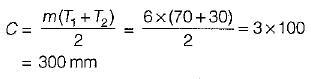QUESTION: 3

### Spot the wrong relation:

Solution:
QUESTION: 4

Which aspect is not true for a stub tooth when compared to full depth system?

Solution:

The interfering portion of the tooth, which is a part of addendum, is removed in stub tooth profile because stub involute system have shorter addendum and dedendum.

QUESTION: 5

Lewis equation in spur gears is applied

Solution:

The amount of force that can be transmitted to a gear tooth is a function of the Soy product as shown by the Lewis equation. For two mating gears, the weaker will have the smaller S0y value. When two mating gears are to be made of .the same material, the smaller gear (pinion) will be the weaker and control design.

QUESTION: 6

Allowable tooth stress for gear-tooth design depends upon the following parameters:
1. Type of gear material
2. Pitch line velocity
3. Beam strength of gear teeth

Which of the above is/are valid?

Solution:

Allowable stress,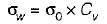where,

σ0 is the endurance strength of material
Cv is the velocity factor

QUESTION: 7

The tooth profiles must not have excessive contact stress as determined by the wear load to insure durability of a gear pair. This wear load is obtained using

Solution:

Buckingham equation,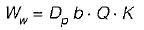where,

Ww = Maximum or limiting load for wear, N
Dp = PCD, mm
b = face width of the pinion , mm
Q = Ratio Factor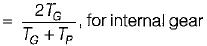K = Load-stress factor or material combination factor, N/mm2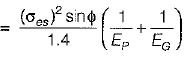where,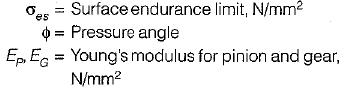QUESTION: 8

A pair of straight teeth spur gears with 6 mm module, having 20° involute full depth teeth is to transmit 12 kW at 300 rpm of the pinion. The speed ratio is 3:1. The allowable static stresses for gear of cast irion and pinion of steel are 60 MPa and 105 MPa respectively.
Assume the following:
Number of teeth on pinion = 16;
Face width = 14 m;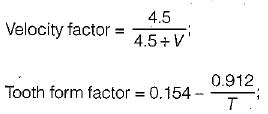σes - 600 MPa; Ep = 200 GPa; EG = 100 GPa; Service factor, Cs = 1; sin20° = 0.342.
Based on these design data, match the followings: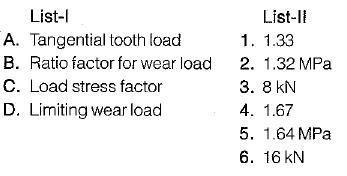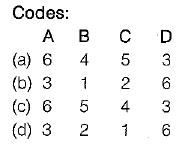Solution:

Pitch line velocity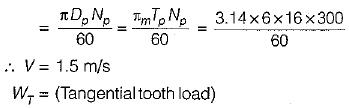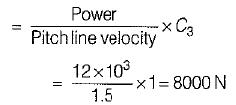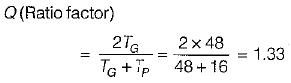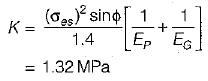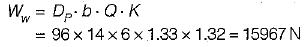QUESTION: 9

The backlash for spur gears depends upon

Solution:
QUESTION: 10

Cycloidal tooth profile of a cycloidal gear tooth is a combination of

Solution:

Cycloidal tooth is made of two curves- hypocycloid curve below the pitch circle and epicycloid curve above the pitch curve.